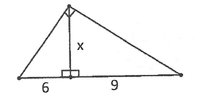#### Cheema

##### New member
Can anyone explain this math problem to me? How would I solve for “x”? I know that I have a large Right Triangle which has been bisected on its Right Angle to create two, smaller Right Triangles. Since each (of these smaller triangles) would then have two angles measuring 45 Degrees, doesn't it mean that I’m dealing with two 45-45-90 Triangles (the legs of the triangles are equal)? Based on this reasoning, I’m confronted by a conundrum because that gives me two values for “x”, 9 & 6 — which is obviously an error. Could anyone more apt at math than myself help me grasp the solution to this problem?#### lev888

##### Full Member
Please explain how you got the 2 45 degree angles.

#### Dr.Peterson

##### Elite Member
The right ange is not bisected; the line is an altitude, not an angle bisector.

Look for similar triangles.

#### pka

##### Elite Member
Can anyone explain this math problem to me? How would I solve for “x”?
View attachment 16630
If you can establish that the two smaller triangles are similar then you can see that $$\dfrac{6}{x}=\dfrac{x}{9}$$.
There is a theorem to that effect:
In any right triangle the altitude from the right angle to the hypotenuse is a mean proportional between the 'parts'.

#### Jomo

##### Elite Member
Yes, if the two angles are both 45, then you have a 45-45-90 triangle. However just because you drop a perpendicular line from a right angles does not mean the other two angles are 45 and 45. Think about this. Suppose you have a right triangle where the angles are 30-60-90. Now you drop a perpendicular line from the right angles. Do the 30 and 60 degree angles magically become both 45 degrees? Please think about this!!

#### Jomo

##### Elite Member
I would 1st label the 4 angles with the fewest number of variables as I can. Then see if the triangles are similar. If they are then follow pka's post #4

#### Kris76

##### New member
Since main triangle is a right-angled and X is altitude andknown segments 6 and 9 the easiest way to find X is X^2=6 x 9 ot X=sqrt(54) or X = app.7.35

#### Jomo

##### Elite Member
How did you conclude that x^2 = 9*6?